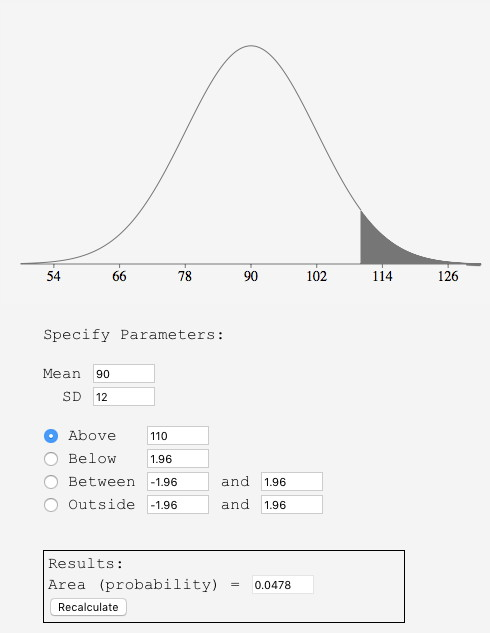# 7.3: Areas Under Normal Distributions

•• David Lane
• Rice University
$$\newcommand{\vecs}{\overset { \rightharpoonup} {\mathbf{#1}} }$$ $$\newcommand{\vecd}{\overset{-\!-\!\rightharpoonup}{\vphantom{a}\smash {#1}}}$$$$\newcommand{\id}{\mathrm{id}}$$ $$\newcommand{\Span}{\mathrm{span}}$$ $$\newcommand{\kernel}{\mathrm{null}\,}$$ $$\newcommand{\range}{\mathrm{range}\,}$$ $$\newcommand{\RealPart}{\mathrm{Re}}$$ $$\newcommand{\ImaginaryPart}{\mathrm{Im}}$$ $$\newcommand{\Argument}{\mathrm{Arg}}$$ $$\newcommand{\norm}{\| #1 \|}$$ $$\newcommand{\inner}{\langle #1, #2 \rangle}$$ $$\newcommand{\Span}{\mathrm{span}}$$ $$\newcommand{\id}{\mathrm{id}}$$ $$\newcommand{\Span}{\mathrm{span}}$$ $$\newcommand{\kernel}{\mathrm{null}\,}$$ $$\newcommand{\range}{\mathrm{range}\,}$$ $$\newcommand{\RealPart}{\mathrm{Re}}$$ $$\newcommand{\ImaginaryPart}{\mathrm{Im}}$$ $$\newcommand{\Argument}{\mathrm{Arg}}$$ $$\newcommand{\norm}{\| #1 \|}$$ $$\newcommand{\inner}{\langle #1, #2 \rangle}$$ $$\newcommand{\Span}{\mathrm{span}}$$

Learning Objectives

• State the proportion of a normal distribution within $$1$$ and within $$2$$ standard deviations of the mean
• Use the calculator "Calculate Area for a given $$X$$"
• Use the calculator "Calculate $$X$$ for a given Area"

Areas under portions of a normal distribution can be computed by using calculus. Since this is a non-mathematical treatment of statistics, we will rely on computer programs and tables to determine these areas. Figure $$\PageIndex{1}$$ shows a normal distribution with a mean of $$50$$ and a standard deviation of $$10$$. The shaded area between $$40$$ and $$60$$ contains $$68\%$$ of the distribution.Figure $$\PageIndex{1}$$: Normal distribution with a mean of $$50$$ and standard deviation of $$10$$.

$$68\%$$ of the area is within one standard deviation ($$10$$) of the mean ($$50$$)

Figure $$\PageIndex{2}$$ shows a normal distribution with a mean of $$100$$ and a standard deviation of $$20$$. As in Figure $$\PageIndex{1}$$, $$68\%$$ of the distribution is within one standard deviation of the mean.Figure $$\PageIndex{2}$$: Normal distribution with a mean of $$100$$ and standard deviation of $$20$$.

$$68\%$$ of the area is within one standard deviation ($$20$$) of the mean ($$100$$).

The normal distributions shown in Figures $$\PageIndex{1}$$ and $$\PageIndex{2}$$ are specific examples of the general rule that $$68\%$$ of the area of any normal distribution is within one standard deviation of the mean.

Figure $$\PageIndex{3}$$ shows a normal distribution with a mean of $$75$$ and a standard deviation of $$10$$. The shaded area contains $$95\%$$ of the area and extends from $$55.4$$ to $$94.6$$. For all normal distributions, $$95\%$$ of the area is within $$1.96$$ standard deviations of the mean. For quick approximations, it is sometimes useful to round off and use $$2$$ rather than $$1.96$$ as the number of standard deviations you need to extend from the mean so as to include $$95\%$$ of the area.Figure $$\PageIndex{3}$$: A normal distribution with a mean of $$75$$ and a standard deviation of $$10. 95\%$$ of the area is within $$1.96$$ standard deviations of the mean.

The normal calculator can be used to calculate areas under the normal distribution. For example, you can use it to find the proportion of a normal distribution with a mean of $$90$$ and a standard deviation of $$12$$ that is above $$110$$. Set the mean to $$90$$ and the standard deviation to $$12$$. Then enter "$$110$$" in the box to the right of the radio button "Above." At the bottom of the display you will see that the shaded area is $$0.0478$$. See if you can use the calculator to find that the area between $$115$$ and $$120$$ is $$0.0124$$.Figure $$\PageIndex{4}$$: Display from calculator showing the area above $$110$$.

Say you wanted to find the score corresponding to the $$75^{th}$$ percentile of a normal distribution with a mean of $$90$$ and a standard deviation of $$12$$. Using the inverse normal calculator, you enter the parameters as shown in Figure $$\PageIndex{5}$$ and find that the area below $$98.09$$ is $$0.75$$.Figure $$\PageIndex{5}$$: Display from normal calculator showing that the $$75^{th}$$ percentile is $$98.09$$.

Normal and Inverse Normal Calculator

This page titled 7.3: Areas Under Normal Distributions is shared under a Public Domain license and was authored, remixed, and/or curated by David Lane via source content that was edited to the style and standards of the LibreTexts platform; a detailed edit history is available upon request.